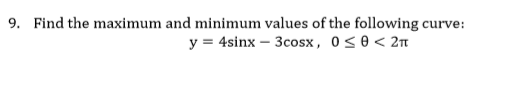# 9.Find the maximum and minimum values of the following curve:

Question
82 viewshelp_outlineImage Transcriptionclose9. Find the maximum and minimum values of the following curve: fullscreen
check_circle

Step 1

To find the maximum and minimum values of the given curve, first we find the critical points.

To find the critical points, we find the first derivative and set that equal to zero and solve for x values.

So we differentiate both sides with respect to x.

We know that the derivative of sin(x) is cos(x)  and the derivative of the cos(x) is -sin(x)

Step 2

Now for critical points, we set the first derivative zero.

Now we simplify further by first subtracting 4 cos(x) both sides and then divide both sides by 3cos(x).

Now on the left side, we use the trigonometric ratio, tan(x)=sin(x)/cos(x)

Step 3

Now to solve for x, we use the concept that if tan(x)=m, then x=arctan(m)

And then using a calcula...

### Want to see the full answer?

See Solution

#### Want to see this answer and more?

Solutions are written by subject experts who are available 24/7. Questions are typically answered within 1 hour.*

See Solution
*Response times may vary by subject and question.
Tagged in

### Trigonometry